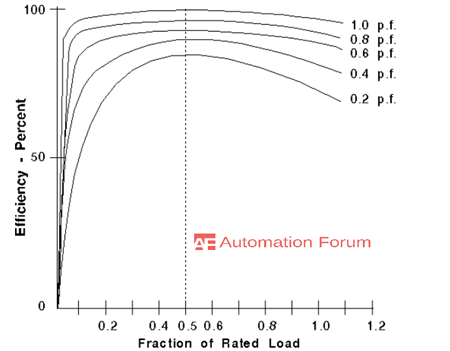# What cause losses in the transformer and how to reduce it ? How to achieve maximum efficiency of a transformer

What is a transformer

A transformer can be considered as the most useful electrical device which has ever invented. It can change the magnitude of alternating current and voltage from one value to another and this useful property of a transformer is the major reason to use alternating current rather than the direct current. So electric power is generated transmitted and distributed in the form of alternating current. There are no moving parts for a transformer and they have high efficiency. The transformer is a device that is used to either raise or lower the voltage of an AC supply with a corresponding increase or decrease in current.

What causes losses in the transformer and how to reduce it

Mostly transformer energy loss is caused by winding and core losses. Transformer losses vary with loads, in order to design an energy-efficient transformer with a lower loss would require a larger core, good-quality silicon steel, or even amorphous steel for the core and thicker wire.

Transformer losses arise from

Winding losses

Current flowing through a winding conductor causes joule heating. When the frequency increases the skin effect and proximity effect causes the winding resistance, and hence increase in loss.

Core loss

The transformer core is crucial in creating the required magnetic circuit. By using a good quality of iron core, we could get a stronger magnetic circuit. The magnetic circuit is the reason for the interlinking of the flux between the windings. Iron loss arises in a transformer as the currents get induced in it while creating the magnetic circuit.

Hysteresis loss

Hysteresis loss is heat loss due to the continuous magnetic field reversal. Energy will be lost by reversing the magnetic field in the core as the magnetizing AC rises and falls and reverses direction. So when each time the magnetic field is reversed a small amount of energy is lost due to hysteresis within the core. We can reduce the hysteresis loss by choosing high-quality magnetic material.

Eddy current loss

Eddy current loss is due to the current flow within the core due to changing magnetic fields. The core has to be suitably designed to control them to the desired levels. In order to reduce the eddy current core can be made by stacking thin sheets together and this will reduce the eddy current. Eddy currents are formed in the metal transformer core and cause heating of the core. The eddy current loss is a complex function of the square of supply frequency and inverse square of material thickness. This loss can be reduced by making the core of a stack of plates electrically insulated from each other.

Stray loss

Stray loss can be considered as the combination of the core loss which is caused by the distortion of an air gap and the I2R loss which is by the non- uniform distribution of conductor current.

Mechanical loss

This type of loss is formed by the bearing friction, brush friction and windage loss and the windage loss includes the power required to circulate air through the machine.

Copper losses

Because of the ohmic resistance copper loss occurs in the primary and secondary windings and it can be checked by a short circuit test. This type of loss is depended upon the magnitude of the current which flows through the winding.

What is the efficiency of a transformer and maximum efficiency of a transformer

Transformer efficiency will increase with an increase in transformer capacity. Like most of the electrical machines, the efficiency of the transformer is defined as the ratio of the output power to the input power.

Power output= power input – total loss, Power input= power output + total losses

Efficiency = power output ÷ power input

The power measured in the primary and secondary windings will be close but it won’t be equal. There will always be a small power loss, which means that not all the energy is transferred from the primary to the secondary winding. The loss of energy is a measure of the transformer’s efficiency. The transformer’s efficiency is the ratio of real output power to real input power. The power rating of the load is the real output power, and the sum of output power plus the transformer loss is the input power.

Condition for maximum efficiency

The efficiency of transformer changes as the function of loading and power factor. The efficiency varies with the load when load increases efficiency increases, the below figure shows the five different efficiency curves for the five different conditions of lagging power factor. Maximum efficiency is achieved at about half load and the maximum efficiency at half load is attained by adjusting a balance between specific design variables related to magnetic core and conductors.• When there is no load, there won’t be any losses and the efficiency is zero because power out is zero.
• Efficiency increase when there is a small application of load
• Mostly maximum efficiency occurs at one-half load
• For the same value of load current, efficiency decreases slightly with decreasing values of power factor, and at the same load point efficiency will be maximum